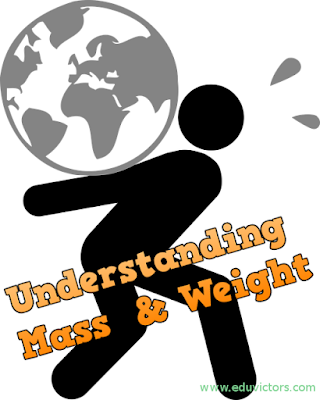## Understanding Mass and Weight

Physics - Gravitation

Mass and Weight
The quantity of matter in a body is known as mass of the body. Mass is quantitative measure of inertia. Mass is an intrinsic property of matter and does not change as an object is moved from one location to another. Weight, in contrast, is the gravitational force that earth exerts on the object and can vary, depending on how far the object is above the earth's surface or whether it is located near another body such as the moon. The relation between weight W and mass m can be written in one of two ways:

W = GMₑm / r²
W = mg

According to the Newton's second law,
Force = mass ✕ acceleration

or mass = force / acceleration

Mass is of two types :
i) Inertial mass

ii) Gravitational mass

1. Inertial Mass:
Ratio of the applied external force and the acceleration produced in the body is known as inertial mass,

mi = External force applied / Acceleration produced.

2. Gravitational Mass:
The ratio of the force of gravity on the body and acceleration produced in the body is known as gravitational mass of the body,

mg = Force of gravity / Acceleration produced.

mi and mg have the same magnitude for a body, so mi = mg.

Another Way to define Weight:
The weight of a body is the force with which the body acts on a horizontal support or a vertical suspender due to the gravitational attraction to the earth. This definition is valid for the reference frame fixed to the support or suspender and corresponds to practical determination of weight in terrestrial conditions with the help of a spring balance.

Generally, the weight of a body is the same in all inertial reference systems (acceleration = 0). It is equal in magnitude to the force of gravity. However, these two forces should not be considered identical.

The weight of the body is the force applied to the support or suspender by the body, while the force of gravity is exerted on the body by the earth. In non-inertial reference systems (acceleration ≠ 0), the magnitude of the weight and force of gravity are different.

Q1: When a ball is thrown upwards, it falls back to the ground. Name the force which makes it fall.

Answer: Gravitational force or Force due to gravity.

Q2: Name the scientist who identified the relation about force of gravitation. Name the law.

Answer: Newton. Newton's law of universal gravitation (1665)

Q3: Is mass a scalar quantity?

Q4: Is weight a scalar quantity?

Answer: No. It is a vector quantity.

Q5: Define gravity.

Answer: The force of attraction exerted by the earth on a body is called gravitational pull or gravity.

Q6: Why does one feel giddy while moving on a merry go round ?

Answer: When moving in a merry go round, our weight appears to decrease when we move down and increases when we move up, this change in weight makes us feel giddy.

Q7: What is weightlessness?

Answer: Weightlessness means zero weight. The state of weightlessness (zero weight) can be observed in the following situations:

(1) When objects fall freely under gravity

(2) When a satellite revolves in its orbit around the earth

(3) When bodies are at null points in outer space. The zero gravity region is called null point.

Q8: The tangential velocity of a planet or moon moving in a circle is at a right angle to ____________. (fill in the blank)